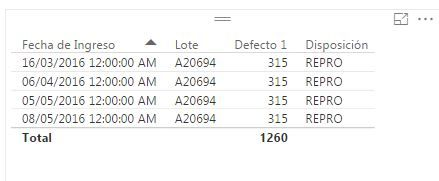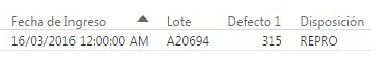cancel
Showing results for
Did you mean:Helper III

## Filter Query (or create new table) based on first (date) duplicate and values of 2 columns

Hello Everyone,
I've tried my best to find the best solution for my issue on the forums, but got into a dead end, so im asking for help from scratch. instead of modifying my attempts, here it goes:
I have a defect table and intend to filter my query or create a new table acording the following criteria:

First date of defect, no duplicate batch#, Process = Tinto , State <> Aprobado

Table for reference:Thank You

1 ACCEPTED SOLUTIONMicrosoft

Aha, I think I follow now

```Table 3 =
VAR T1 = SUMMARIZE('Table','Table'[BATCH#],"First Date" , MIN('Table'[DATE]))
VAR T2 = FILTER(
NATURALINNERJOIN('Table',T1),[STATE]<> "Aprobado" && [PROCESS]="Tinto" && [First Date] = [DATE])
RETURN T2```

Proud to be a Datanaut!

7 REPLIES 7Microsoft

Im not sure if I fully understand your needs but this is my interpretation.  Let me know how close I am.

```Table 2 =

var T1 = FILTER(
'Table',
'Table'[PROCESS]="Tinto"
)

var T2 = SUMMARIZE(
T1,
'Table'[BATCH#] ,
"Max Date" ,
MAX('Table'[DATE]))
return NATURALINNERJOIN(T1,T2)```

Proud to be a Datanaut!Helper III

Hello Phil,

This is close but not exactly what I'm looking for, this is my logical thinking of how it should happen:

First I need to find the earliest a batch is in the data, no matter if it complies with the other filtered criteria, afterwards it should filter only leave on the table the ones that comply.

Basically im looking for all batches that in their first input comply with the criteria status<> "aprobado" and process  ="Tinto".

This is what i got with your suggested formula:They comply with the filters but have duplicate batch #.

I hope I explained myself properly this time.

Any more thougths?

ThanksMicrosoft

Is this getting closer?

```Table 3 =
VAR T1 = SUMMARIZE('Table','Table'[BATCH#],"First Date" , MIN('Table'[DATE]))
VAR T2 = FILTER(
RETURN SUMMARIZE(T2,'Table'[BATCH#] , "Batches" , COUNTROWS('Table') , "First Date" , min('Table'[DATE]))```

Proud to be a Datanaut!Microsoft

Can you please post a mock-up of what the result table should look like?

Proud to be a Datanaut!Helper III

Same as the one in my last post but only with the top result which is the ealiest date:Microsoft

Aha, I think I follow now

```Table 3 =
VAR T1 = SUMMARIZE('Table','Table'[BATCH#],"First Date" , MIN('Table'[DATE]))
VAR T2 = FILTER(
NATURALINNERJOIN('Table',T1),[STATE]<> "Aprobado" && [PROCESS]="Tinto" && [First Date] = [DATE])
RETURN T2```

Proud to be a Datanaut!Helper III

Thank you!